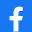# Capital Budgeting (Theory)

REALIZAR TEST
 Título del test: Capital Budgeting (Theory) Descripción: Brief review Autor:Jesús H. Escárcega(Otros tests del mismo autor)Fecha de Creación: 13/01/2021 Categoría: Otros Número preguntas: 27
 Comparte el test:REALIZAR TEST
 Últimos Comentarios
No hay ningún comentario sobre este test.
Temario:
 Should a firm invest in projects with NPV = \$0? Yes No The firm should look at the PI and IRR of the projects The firm is indifferent between accepting or rejecting projects with zero NPVs .The preferred technique for evaluating most capital investments is: Payback period Net present value Internal rate of return Discount payback period.The capital budgeting process involves: Identifying potential investments Others are correct Analyzing the set of investment opportunities, and identifying those that will create shareholder value Implementing and monitoring the selected investment projects .A project may have multiple IRRs when: The project generates an alternating series of net cash inflows and outflows. The project generates an immediate cash inflow followed by cash outflow. The project has a negative NPV. The project is of considerably large scale.The IRR method assumes that the reinvestment rate of cash flows is: The cost of capital. The hurdle rate. The IRR Zero.You must know the discount rate of an investment project to compute its: NPV, IRR, PI, and discount payback period NPV, PI , discount payback period NPV, PI, IRR .You must know all the cash flows of an investment project to compute its: NPV, IRR, and discount payback period. NPV, IRR, payback period, and discount payback period. NPV, IRR. NPV, accounting rate of return, IRR.The figure shows the NPV profile for two investment projects. What’s the IRR for project B? 10% 15% 25%.The figure shows the NPV profile for two investment projects. If projects are mutually exclusive and if the discount rate was 5%, which project should be chosen? A B.The figure shows the NPV profile for two investment projects. If projects are mutually exclusive and if the discount rate was 20%, which project should be chosen? A B.If projects are independent and if the discount rate was 5%, which projects should be chosen? A B BOTH OF THEM.The figure shows the NPV profile for two investment projects. If projects are mutually exclusive and if the discount rate was 10%, which project should be chosen? A B IS INDIFFERENT.If projects are independent and if the discount rate was 10%, which projects should be chosen? A B BOTH OF THEM IS INDIFFERENT.If projects are independent and if the discount rate was 20%, which projects should be chosen? A B NEITHER OF THE TWO BOTH OF THEM IS INDIFFERENT.If projects are independent and if the discount rate was 30%, which projects should be chosen? A B BOTH OF THEM NEITHER OF THE TWO.The process of identifying which long-lived investment projects a firm should undertake is known as: capital spending capital budgeting capital hedging capital investment. .Capital budgeting techniques should recognize the time value of money. False True. The main virtue of the payback method is its: simplicity complexity completeness thoroughness. .The payback method: fails to explicitly consider the time value of money. is the amount of time it takes for a project to recoup its profits. is the best method for evaluating complex projects. is never used by businesses today. .A problem with the payback method is: it assigns a 0 percent discount rate to cash flows that occur before the cutoff point. it assigns a 1 percent discount rate to cash flows that occur before the cutoff point. it assigns a 10 percent discount rate to cash flows that occur before the cutoff point. it assigns a 100 percent discount rate to cash flows that occur before the cutoff point. .As the discount rate increases, the NPV of a project: increases. decreases. is unaffected. cannot be determined with out knowing the discount rate.As the discount rate increases, the IRR of a project: increases. decreases. is unaffected. cannot be determined with out knowing the discount rate. .The NPV method focuses on: sales. accounting returns. profits. cash flows. .When the IRR is equal to the discount rate, the NPV is: positive. equal to zero. negative. cannot be determined without knowing the discount rate. .Is a problem with the Internal Rate of Return? multiple IRRs very complex calculation accounting method.When net present value is positive: the internal rate of return equals the cost of capital. the internal rate of return exceeds the cost of capital. the internal rate of return is less than the cost of capital. the internal rate of return equals zero. .The discount rate that equates present value of cash inflows and outflows is called the: component cost of capital. weighted average cost of capital after-tax weighted average cost of capital. IRR.
 Denunciar test Condiciones de uso
INICIO
CREAR TEST
INFORMACIÓN